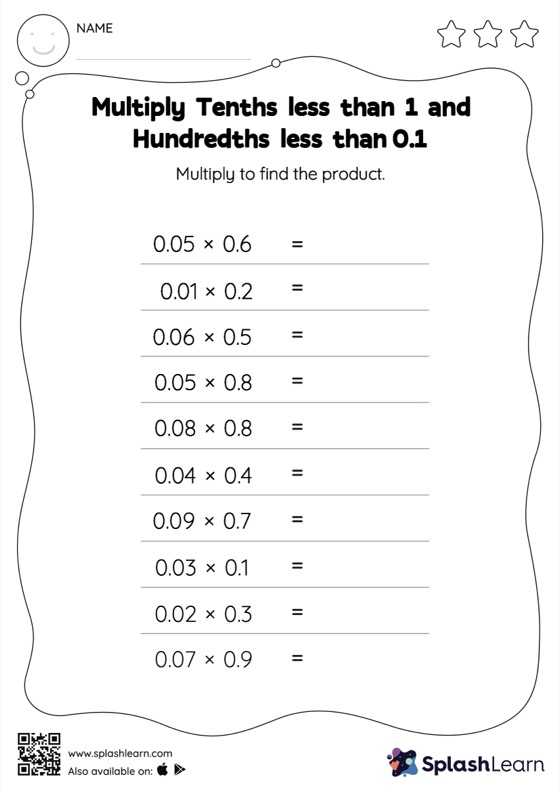# Multiply Tenths less than 1 and Hundredths less than 0.1: Horizontal Multiplication Worksheet

Home > Multiply Tenths less than 1 and Hundredths less than 0.1: Horizontal MultiplicationGive your little one some practice building multiplication skills with this multiply tenths less than 1 and hundredths less than 0.1 Worksheet. This worksheet is about practicing with the horizontal format in which numbers are written side by side. To develop flexibility with numbers and operations, students need to have enough practice in this format and not just rely on the vertical/column method.